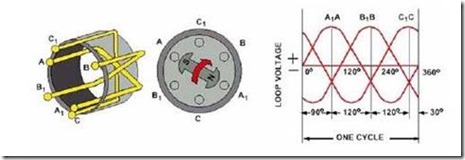Home > English > Various Kinds Of Alternator

# Various Kinds Of Alternator

In Figure 16 a single wire / wire node acting as a stator winding and a magnetic rod acting as a rotor illustrates how the AC voltage / voltage is produced within a basic alternator. If two wire / wire nodes again, which are 120 degrees apart, are added to our base alternator, then two separate seperate voltages will be produced.Figure 1.16

With the S pole of the rotor directly below the conductor A, then the voltage / voltage at A will be at the maximum and positive quantity in the polarity. After the rotor rotates 120 degrees, then the S pole will be directly below conductor B and the voltage / voltage at B will be a positive maximum. Also 120 degrees later, the voltage / voltage on C will be a positive maximum. The peak positive voltage / voltage at A, B, C inside each wire / wire node occurs at a distance of 120 degrees. The voltage / vertex knot is also shown in the graph above.

If the ends of the wire / wire nodes, marked A1, B1 and C1, are connected to the ends marked B, C and A, then a three-stage “delta” groundwind stator will be formed (Fig. 19). The three AC (BA, CB and AC) voltages available from the delta stator windings are the same as the three voltages discussed earlier.

B. Alternator winding star / YFigure 1.18

If the ends of the wire nodes / wires marked A1, B1 and C1 are connected together, a basic three-stage winding stator “Y” will be formed (Fig. 20). Each of these voltages / voltages consists of the voltage / voltage within the two wire nodes / wires that are added together. Three AC voltages with 120-degree distances are available from stator Y. Stator Y is often called a star configuration.

In the delta windings, each individual winding is connected at the other end of the coil (Fig. 18). This creates a parallel relationship within the delta stator, which generally allows a higher current output than the “Y” winding stator. In the stator winding “Y”, the coil is connected to form a series relationship. This series of relationships generally provide a higher voltage / voltage but a lower current output than the delta winding stator. Current alternator manufacturers use both “delta” and “Y” coils in the stator.

To increase the output of the alternator some modifications on the basic model are required by:

•  increase the number of conductors in each winding phase
•  increase the strength of the magnetic field
•  increase spinning speed."Tasikmalaya, Indonesia"